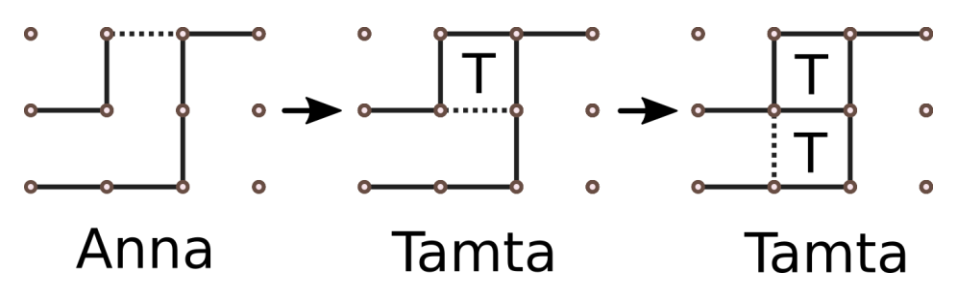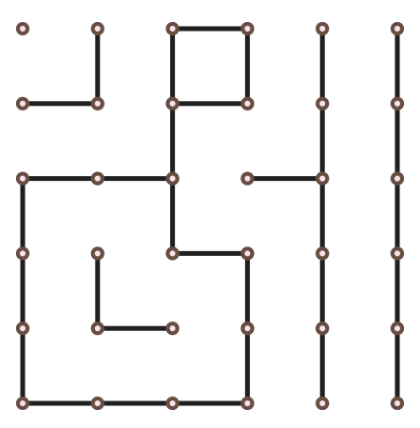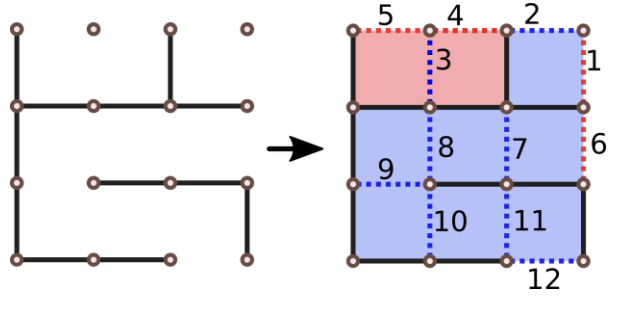시간 제한 메모리 제한 제출 정답 맞은 사람 정답 비율
0.5 초 512 MB 0 0 0 0.000%

## 문제

Tamta and Anna are sisters who like playing a game of Dots and Boxes.

The game starts with an empty N + 1 by M + 1 grid of dots (and correspondingly, N by M grid of boxes). Players take turns adding a single horizontal or vertical edge between two unjoined adjacent dots (two dots are adjacent if the distance between them is 1). If a player completes the fourth side of a 1 × 1 box on her turn she claims it, earns one point and takes another turn, otherwise the turn switches to another player.

The game ends when no more edges can be placed. Possible next three turns in a grid with N = 2, M = 3 (dotted lines are player moves):Anna and Tamta have been playing for a while and you’ve noticed that in a current state each box has exactly zero or two unjoined sides and it's Anna's turn to move. (you can see an example in the image on the right. Note that image above does not fit this description).

The score of this game will be calculated as SA − ST where SA is the number of points Anna earns from this point forward and ST is the number of points that Tamta earns. Obviously, Anna is trying to maximize the score while Tamta is trying to minimize it. You need to calculate the final score knowing both players act optimally.

## 입력

The first line contains two integers N and M which are number of rows and number of columns in a grid of boxes.

Each of the next N + 1 lines contain M digits, each being one or zero (without separating spaces), j-th number in the i-th row is one if and only if there is an horizontal edge between the dots with coordinates (i,j) and (i,j+1).

Following N lines contain M + 1 digits in the same format, j-th number in i-th row is 1 if and only if there is a b edge between the dots with coordinates (i,j) and (i+1,j).

## 출력

The only line should contain a single integer, the final score.

## 제한

• 3 ≤ N, M ≤ 20
• each box has exactly zero or two unjoined sides

## 예제 입력 1

3 3
000
111
011
110
1010
1000
1001


## 예제 출력 1

-5


## 예제 입력 2

5 5
00100
10100
11010
00100
01000
11100
011111
001011
101011
110111
100111


## 예제 출력 2

6


## 힌트

The first example and one of the possible optimal move orders are depicted below (numbers on edges indicate turn order, red colors used by Anna and blue used by Tamta).

Second example is the one shown in the above pictures.